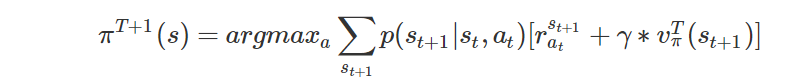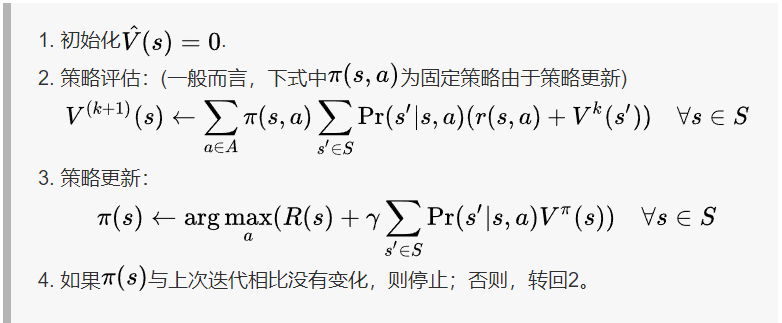# 吴恩达Stanford机器学习公开课（十六）笔记

## Lecture 16 - Reinforcement Learning and Control

Posted by Yunlongs on June 26, 2019

# Lecture 16 - Reinforcement Learning and Control

## Markov decision processes (MDP)

• S是状态的集合
• A是动作的集合
• $P_{sa}$是状态转移概率
• $\gamma \in[0,1)$是折扣因子
• $R : S \times A \mapsto \mathbb{R}$是反馈函数

MDP处理中的属性变化如下： 从状态$s_0$开始，选择一些动作$a_{0} \in A$来进行MDP。作为结果，MDP的状态会随机的变化到下一个状态$s_1$，其中$s_{1} \sim P_{s_{0} a_{0}}$。然后，我们选择另一个动作$α_1$，状态再一次的改变，现在变成了状态$s_{2} \sim P_{s_{1} a_{1}}$。。。。

Bellman等式可以有效的去解出$V^π$ ， 特别是在有限状态集MDP中，我们可以为其中的每一个特状态s都写出其等式$V^{\pi}(s)$，这个等式会产生$|S|$个新的等式，把这些等式当做为方程组（$|S|$个变量和$|S|$个方程组），就可以被高效的解出。

BEllman等式 版本的最优价值函数为其中第一项仍然是之前的即时反馈，第二项是 在第一步后的所有动作的未来折扣反馈的最大值（相当于多了一层对动作a的循环，并取其最大值）。

## 价值(value)迭代和政策(policy)迭代

1. 对于每个状态s，初始化为$V(s) :=0$
2. 重复直到收敛｛

对于每个状态，做如下更新$V(s) :=R(s)+\max _ {a \in A} \gamma \sum_{s^{\prime}} P_{s a}(s^{\prime}) V(s^{\prime})$

1.这个算法可以被看作为 重复的尝试去使用Bellman等式来更新估计的价值函数。

https://www.cnblogs.com/huangyc/p/10381184.html因此，内层的循环将会重复地为当前的政策计算价值函数,直至价值收敛，然后使用当前的价值函数来更新政策,直至政策收敛，然后再使用所得的政策重新进行步骤（2） （在步骤（3）中要寻找的$π$也被称作关于$V$的贪婪政策）。注意这里步骤(2)可以使用Bellman等式来解出，对于一个固定的政策需要计算$|S|$个变量组成的$|S|$的线性方程组。

## DMP的模型学习

1. 随机初始化$π$
2. 重复｛

(a) 在MDP中执行$π$来进行多个试验

(b) 使用在MDP中积累的经验，来更新我们对$P_{sa}$的估计(如果可以的话，还有R)

(c) 根据估计的模型参数，应用价值迭代来得到新的价值函数V的估计

(d) 更新$π$使其成为越来越贪婪的政策 ｝ 注意到在这个算法中做了一些小改进，在内层的价值迭代中并没有初始化V=0，而是将其初始化为前一轮迭代的解，这将会为价值迭代提供一个更好的起始点，并能够更快速的收敛